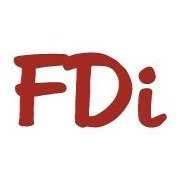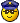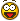# 3d polyline with elevation gained from a 3d face

## Recommended PostsHi all,

I have surveyed some pipes below the ground and I need to represent these as 3d polylines in Autocad below a ground surface model.

Is there a routine that will let me add a z value to each vertex along a 3d polyline line which references a 3d face/ground model above to give me a true z value directly below the ground level.

Any Ideas???

##### Share on other sitesSince both use WCS points exclusively, you should be able to come to a close approximation of the Z axis values. With NON Coplaner faces, you will not be able to determine an exact value due to fact that autocad does not have a way to determine which 3 points of the face makes a plane. -David

##### Share on other sitesIf I understand correctly... try this```
(defun c:test (/ os lup list_V 3DP V_coord 3DF_tot P_verif pINT_z)
;;; GP 07. May. 2012 ;;;

(setq olderr  *error*  *error* myerror)
(if (not (equal (getvar "viewdir") (list 0.0 0.0 1.0)))
(progn
"Set \"View\" \"_Top\" "
"\nbefore launching the lisp."
)   )
(exit)
)
)
(if (not (member "geomcal.arx" (arx))) (arxload "geomcal"))
(if
(and
(setq 3DP (vlax-ename->vla-object (car (entsel "\nSelect 3Dpoly"))))
(= (vlax-get 3DP 'ObjectName) "AcDb3dPolyline")
)
(progn
(command "_undo" "_begin")
(setq lup (getvar "luprec"))
(setvar "luprec" 2)
(command "_ucs" "_w")

(setq V_coord (vlax-get 3DP 'Coordinates))
(repeat (/ (length V_coord) 3)
(setq V_coord (cdddr V_coord))
)
(command "_ZOOM" "_OBJ" (vlax-vla-object->ename 3DP) "")
(setq 3DF_tot (ssget "_F" list_V '((0 . "3DFACE"))))
(command "_ZOOM" "_P")
(if (= 3DF_tot nil)
(progn
(exit)
)
)
(repeat (length list_V)
(setq P_verif (car list_V))
(PUNTO_INTERNO)
(if (/= pINT_z nil)
(entmake
(list
(cons 0 "TEXT")
(cons 7 (getvar "textstyle"))
(cons 10 P_verif)
(cons 40 (getvar "textsize"))
(cons 1 (rtos (- (last P_verif)(last pINT_z))))
)
)
)
(setq list_V (cdr List_V))
)

(setvar "luprec" lup)
(command "_ucs" "_p")
(command "_undo" "_end")
(prompt "\n ") (prompt "\n ")
(princ)
)
)
)

;;;;;;;;;;;;;;;;;;;;;;;;;;;;;;;;;;;;;;;;;;;;;;;;;;;;;;;;;;;;;;;;;;;;;;
;              occurs if the point is inside the 3DFACE
;         return pINT_z (internal point of intersection 3DFACE)
;               data: P_verif -> point to test
;                     3DF_tot -> group of 3DFACE
(defun PUNTO_INTERNO (/ 3df pFIN P_INTERNO N_verificate e1 p1 p2 p3 ang_p1p ang_p1_2
ang_p1_3 ang_p2p ang_p2_1 ang_p2_3 ang_p3p ang_p3_1 ang_p3_2
ang_p1- ang_p1+ ang_p2- ang_p2+ ang_p3- ang_p3+ COMPR)

(setq 3df -1)
(setq pINT_z nil)
(setq P_INTERNO T)
(setq pFIN P_verif)
(setq N_verificate 0)
(while P_INTERNO
(setq e1 (ssname 3DF_tot (setq 3df (1+ 3df))))
(setq p1 (cdr (assoc 10 (entget e1))))
(setq p2 (cdr (assoc 11 (entget e1))))
(setq p3 (cdr (assoc 12 (entget e1))))
(setq ang_p1p (angle p1 pFIN))
(setq ang_p1_2 (angle p1 p2))
(setq ang_p1_3 (angle p1 p3))
(setq ang_p2p (angle p2 pFIN))
(setq ang_p2_1 (angle p2 p1))
(setq ang_p2_3 (angle p2 p3))
(setq ang_p3p (angle p3 pFIN))
(setq ang_p3_1 (angle p3 p1))
(setq ang_p3_2 (angle p3 p2))
(setq ang_p1- (min ang_p1_2 ang_p1_3))
(setq ang_p1+ (max ang_p1_2 ang_p1_3))
(setq ang_p2- (min ang_p2_1 ang_p2_3))
(setq ang_p2+ (max ang_p2_1 ang_p2_3))
(setq ang_p3- (min ang_p3_1 ang_p3_2))
(setq ang_p3+ (max ang_p3_1 ang_p3_2))
(setq COMPR 0)
(if (and (< ang_p1- (/ pi 2)) (> (- ang_p1+ ang_p1-) pi))
(progn
(if (or (> ang_p1p ang_p1+) (< ang_p1p ang_p1-))
(setq COMPR (1+ COMPR))
)
)
)
(if (and (< ang_p2- (/ pi 2)) (> (- ang_p2+ ang_p2-) pi))
(progn
(if (or (> ang_p2p ang_p2+)(< ang_p2p ang_p2-))
(setq COMPR (1+ COMPR))
)
)
)
(if (and (< ang_p3- (/ pi 2)) (> (- ang_p3+ ang_p3-) pi))
(progn
(if (or (> ang_p3p ang_p3+)(< ang_p3p ang_p3-))
(setq COMPR (1+ COMPR))
)
)
)
(if (and (> ang_p1p ang_p1-) (< ang_p1p ang_p1+)) (setq COMPR (1+ COMPR)))
(if (and (> ang_p2p ang_p2-) (< ang_p2p ang_p2+)) (setq COMPR (1+ COMPR)))
(if (and (> ang_p3p ang_p3-) (< ang_p3p ang_p3+)) (setq COMPR (1+ COMPR)))
(if (= COMPR 3)
(progn
(setq p_INT_inf (list (car P_verif) (cadr P_verif) -10000))
(setq p_INT_sup (list (car P_verif) (cadr P_verif) 10000))
(cal "pINT_z=ilp(p_INT_inf,p_INT_sup,p1,p2,p3)")
(setq P_INTERNO nil)
)
)
(setq N_verificate (1+ N_verificate))
(if (= N_verificate (sslength 3DF_tot))(setq P_INTERNO nil))
)
)

(defun myerror (s)
(if (/= s "Function cancelled")
(princ (strcat "\nError: " s))
)
(setvar "luprec" lup)
(command "_ucs" "_p")
(command "_undo" "_end")
(princ)
)
```

##### Share on other sitesewock76.... disappeared##### Share on other sitesewock76.... disappearedInto thin air : Just like him came in##### Share on other sitesHi GP_/anyone,

I am in similar need;

my setup is a mesh made up of 3DFACEs, with 2D polylines overlaid.

I would ideally like to select a 2d poly, and an interlolated level from the 3d mesh would be applied to each vertex of the 2d polyline, effectively turning it into a 3d polyline.

Thank you.

##### Share on other sitesAnother way around this is to explode the 3 dfaces into lines the pline on top becomes a "fence" selection set then you use the "inters" function to find the 3d intersection point and build a new 3d pline from these points.

Simpler way its a function of CIV3d.

Just thinking aloud convert your 3d faces to lines (Will try to find lisp) draw pline trim all new lines to new pline, erase pline and redraw to end of cut lines this is a true 3d pline with z's.

##### Share on other sitesThanks,

I can use the LISP from GP_ after a fashion; I have to draw a 3dPOLY over the top of the 2d line, select it using the routine; this puts text values that are the difference between the mesh & the line;

I then edit each vertex with this level, adjusted to show the pipes depth; a bit of manual labour but it gets me what i want until a better routine is discovered!

##### Share on other sitesI did not understand... maybe this? Or attach a sample dwg.

## Join the conversation

You can post now and register later. If you have an account, sign in now to post with your account.
Note: Your post will require moderator approval before it will be visible.×   Pasted as rich text.   Paste as plain text instead

Only 75 emoji are allowed.